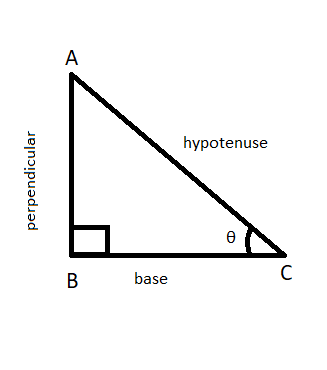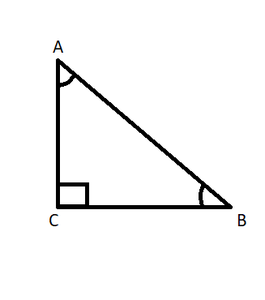# If A and B are acute angles such that cosA = cosB, then prove that A = B

• Last Updated : 22 Sep, 2021

Trigonometry is basically the study of the relationship between the angles and the sides of a triangle. It is one of the widely used topics of Mathematics that is used in daily life. It involves operations on a right-angled triangle i.e. a triangle having one of the angles equal to 90°. There are some terms that we should know before going further. These terms are,

1. Hypotenuse – It is the side opposite to the right angle in a right-angled triangle. It is the longest side of a right-angled triangle. In Figure 1, side AC is the hypotenuse.
2. Perpendicular – The perpendicular of a triangle, corresponding to a particularly acute angle θ is the side opposite to the angle θ. In Figure 1, side AB is the perpendicular corresponding to angle θ.
3. Base – It is the side adjacent to a particularly acute angle θ. In Figure 1 side BC is the base corresponding to angle θ.Figure 1

As earlier said, trigonometry depicts the relationship between the angles and sides of a right-angled triangle. This relationship is represented by standard ratios and is given as follows

Attention reader! All those who say programming isn't for kids, just haven't met the right mentors yet. Join the  Demo Class for First Step to Coding Coursespecifically designed for students of class 8 to 12.

The students will get to learn more about the world of programming in these free classes which will definitely help them in making a wise career choice in the future.

• Sine (sin) – The sine of an angle θ is the ratio of the length of the perpendicular, corresponding to the angle θ, to the length of the hypotenuse of the triangle.

sinθ = perpendicular/hypotenuse = p/h

• Cosine (cos) – The cosine of an angle θ is the ratio of the length of the base, corresponding to the angle θ, to the length of the hypotenuse of the triangle.

cosθ = base/hypotenuse = b/h

• Tangent (tan) – The tangent of an angle θ is the ratio of the length of the perpendicular, corresponding to the angle θ, to the length of the base for the particular angle of the triangle.

cosθ = base/hypotenuse = b/h

• Cotangent (cot) – It is the reciprocal of the tangent.

cotθ = 1/tanθ = base/perpendicular = b/p

• Secant (sec) – It is the reciprocal of cosine.

secθ = 1/cosθ = hypotenuse/base = h/b

• Cosecant (cosec) – It is the reciprocal of sine.

cosecθ = 1/sinθ = hypotenuse/perpendicular = h/p

### If A and B are acute angles such that cosA  = cosB, then prove that A = B

Solution:

Given,

cos A = cos B

Suppose the triangle looks something like the figure 2, with acute angles A and B.Figure 2

Then cos A = AC/AB  ⇢   (i)

cos B = BC/AB  ⇢  (ii)

Since, cos A = cos B

Therefore,

AC/AB = BC/AB

For the equation to be correct, AC should be equal to BC

=> angle A = angle B      (since angles opposite to equal sides are equal)

### Similar Questions

Question 1: If A and B are acute angles such that sin A = sin B then check whether A = B.

Solution:

Given,

sin A = sin B

Suppose the triangle looks something like the figure 2, with acute angles A and B.

Then sin A = BC/AB  ⇢  (i)

sin B = AC/AB  ⇢  (ii)

Since, sin A = sin B

Therefore,

BC/AB = AC/AB

For the equation to be correct, AC should be equal to BC

=> angle A = angle B      (since angles opposite to equal sides are equal)

Question 2: If A and B are acute angles such that tan A = tan B then check whether A = B.

Solution:

Given,

tan A = tan B

Suppose the triangle looks something like the figure 2, with acute angles A and B.

Then tan A = BC/AC  ⇢  (i)

tan B = AC/BC  ⇢  (ii)

Since, tan A = tan B

Therefore,

BC/AC = AC/BC

For the equation to be correct, AC should be equal to BC

=> angle A = angle B      (since angles opposite to equal sides are equal)

Question 3: If A and B are acute angles such that cot A = cot B then check whether A = B.

Solution:

Given,

cot A = cot B

Suppose the triangle looks something like the figure 2, with acute angles A and B.

Then cot A = AC/BC  ⇢  (i)

cot B = BC/AC  ⇢  (ii)

Since, cot A = cot B

Therefore,

AC/BC = BC/AC

For the equation to be correct, AC should be equal to BC

=> angle A = angle B      (since angles opposite to equal sides are equal)

Question 4: If A and B are acute angles such that sec A = sec B then check whether A = B.

Solution:

Given,

sec A = sec B

Suppose the triangle looks something like the figure 2, with acute angles A and B.

Then sec A = AB/AC  ⇢  (i)

sec B = AB/BC  ⇢  (ii)

Since, sec A = sec B

Therefore,

AB/AC = AB/BC

For the equation to be correct, AC should be equal to BC

=> angle A = angle B      (since angles opposite to equal sides are equal)

Question 5: If A and B are acute angles such that cosec A = cosec B then check whether A = B.

Solution:

Given,

cosec A = cosec B

Suppose the triangle looks something like the figure 2, with acute angles A and B.

Then cosec A = AB/BC  ⇢  (i)

cosec B = AB/AC  ⇢  (ii)

Since, cosec A = cosec B

Therefore,

AB/BC = AB/AC

For the equation to be correct, AC should be equal to BC

=> angle A = angle B      (since angles opposite to equal sides are equal)

So, in generic we can say if the trigonometric ratio of one acute angle is equal to the trigonometric ratio of another acute angle then the angles ought to be the same.

My Personal Notes arrow_drop_up